Next: The equation of state Up: Classical thermodynamics Previous: Classical thermodynamics

## Introduction

We have learned that macroscopic quantities such as energy, temperature, and pressure are, in fact, statistical in nature: i.e., in equilibrium they exhibit random fluctuations about some mean value. If we were to plot out the probability distribution for the energy, say, of a system in thermal equilibrium with its surroundings we would obtain a Gaussian with a very small fractional width. In fact, we expect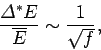(260)

where the number of degrees of freedom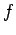is about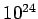for laboratory scale systems. This means that the statistical fluctuations of macroscopic quantities about their mean values are typically only about 1 in.

Since the statistical fluctuations of equilibrium quantities are so small, we can neglect them to an excellent approximation, and replace macroscopic quantities, such as energy, temperature, and pressure, by their mean values. So,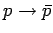, and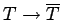, etc. In the following discussion, we shall drop the overbars altogether, so that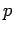should be understood to represent the mean pressure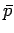, etc. This prescription, which is the essence of classical thermodynamics, is equivalent to replacing all statistically varying quantities by their most probable values.

Although there are formally four laws of thermodynamics (i.e., the zeroth to the third), the zeroth law is really a consequence of the second law, and the third law is actually only important at temperatures close to absolute zero. So, for most purposes, the two laws which really matter are the first law and the second law. For an infinitesimal process, the first law is written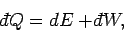(261)

where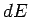is the change in internal energy of the system,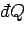is the heat absorbed by the system, and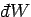is the work done by the system on its surroundings. Note that this is just a convention. We could equally well write the first law in terms of the heat emitted by the system or the work done on the system. It does not really matter, as long as we are consistent in our definitions.

The second law of thermodynamics implies that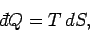(262)

for a quasi-static process, where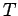is the thermodynamic temperature, andis the change in entropy of the system. Furthermore, for systems in which the only external parameter is the volume (i.e., gases), the work done on the environment is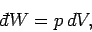(263)

whereis the pressure, and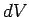is the change in volume. Thus, it follows from the first and second laws of thermodynamics that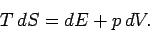(264)Next: The equation of state Up: Classical thermodynamics Previous: Classical thermodynamics
Richard Fitzpatrick 2006-02-02